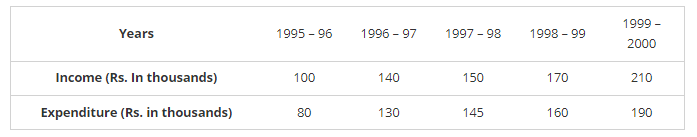# The income and expenditure for 5 years of a family is given in the following data:`
Question:

The income and expenditure for 5 years of a family is given in the following data:

Represent the above data by a bar graph.Solution:

To represent the given data by a vertical bar graph, we first draw horizontal and vertical axes. Let us consider that the horizontal and vertical axes represent the years and the income or expenditure in thousand rupees respectively. We have to draw 5 bars for each income and expenditure side by side of different lengths given in the table.

At first, we mark 5 points for each income and expenditure in the horizontal axis at equal distances and erect rectangles of the same width at these points. The heights of the rectangles are proportional to the corresponding income or expenditures.